# Degenerate elliptic equation

(diff) ← Older revision | Latest revision (diff) | Newer revision → (diff)

A partial differential equation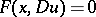(1)

where the real-valued function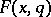satisfies the condition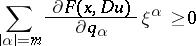(2)

for all real, and there exists afor which (2) becomes an equality. Here,is an-dimensional vector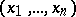;is the unknown function;is a multi-index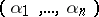;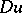is a vector with components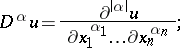the derivatives in equation (1) are of an order not exceeding; the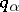are the components of a vector;is an-dimensional vector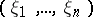; and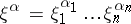. If strict inequality in equation (2) holds for allandand for all real, equation (1) is elliptic at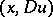. Equation (1) degenerates at the pointsat which inequality (2) becomes an equality for any real. If equality holds only on the boundary of the domain under consideration, the equation is called degenerate on the boundary of the domain. The most thoroughly studied equations are second-order degenerate elliptic equations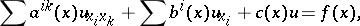where the matrix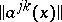is non-negative definite for all-values under consideration.